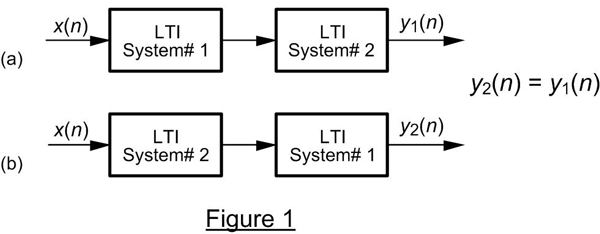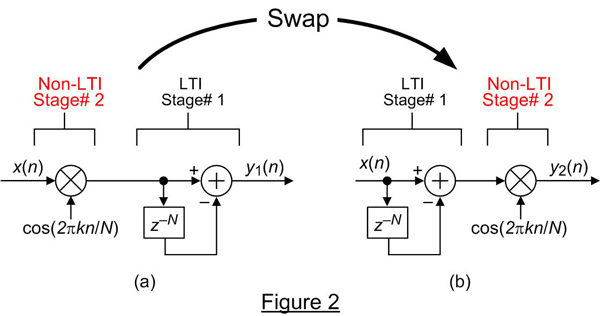# Quiz11

Last updated: June 23rd, 2020

# DSP RELATED (.COM)¶

Linear time-invariant (LTI) systems have a useful commutative property where their sequential order can be rearranged with no change in their final outputs.This situation, described in almost every DSP textbook, is shown in Figure 1 where two different LTI systems are configured in series. Swapping the order of two cascaded LTI systems does not alter the final output. That is, y1(n) is always equal to y2(n).The textbooks don't come right out and say it but they strongly imply that swapping the order of the two systems in Figure 1 is not permitted if either System #1 or System #2 is not LTI.

Now consider the cascaded system, that I encountered recently, shown in Figure 2(a). Variable n is the integer time index, k is a real-valued frequency variable, and N is a positive integer. The multiplication in Stage #2 is not LTI because it is not time-invariant.

### Quiz Question: Under what condition can we swap the order of the stages in Figure 2(a) to create Figure 2(b) such that y1[n] = y2[n]¶

Pick one:

• When k is negative
• When k is an integer
• When 2πk(n-1)/N is aperiodic
• None of the above
In :
import numpy as np
import matplotlib.pyplot as plt
M = 20 # Signal length
mu, sigma = 0, 1
x_n = np.zeros(50)
n = np.arange(-25, 25)  # n is from -25 to 24
x_n[25 - M//2:25 + M//2] =  np.random.normal(mu, sigma, M)  # Random signal from index -10 to 10

In :
def system_1_delay(x, N):
''' Delays input's output
by delay N, AND sums with Input

inputs:
x : input
N : Delay number

output
y : delayed input + input
x[n-N] + x[n]
'''
y = np.zeros(len(x))

if N>0:
y[N:] = x[:-N]
elif N<0:
y[:N] = x[-N:]
else:
y = x[:]

return x + y

In :
def system_2_cos(x, k, N):
''' Multiply input sequence x[n] by cos(2*np.pi*k*n/N)

inputs:
x : input
k : real-valued frequency variable
N : Integer

output
y : delayed input
x[n-N]
'''
N = int(N) # Ensure Integer
n = np.arange(-25, 25)
y = x * np.cos(2*np.pi*k*n/N)
return y

In :
def show_outputs(k, N):
fig, ax = plt.subplots(1,1, figsize=(16,6))
ax.stem(n, x_n, use_line_collection = True)
ax.set(xlabel='n', title='x[n]')
fig, ax = plt.subplots(1,1, figsize=(16,6))
plt.cla()

ax.stem(n, system_1_delay(system_2_cos(x_n, k, N), N),
linefmt ='b', markerfmt= 'bo', use_line_collection = True, label='Fig2-a')
ax.stem(n+0.2, system_2_cos(system_1_delay(x_n, N), k, N),
linefmt ='r', markerfmt= 'ro', use_line_collection = True, label='Fig2-b')
ax.set_title(''.join(['k= ' , str(k), ', N= ', str(N) , ',  k/N= ', str(k/N)]))
ax.set_xlabel('n')
#     print(k,  N)
ax.legend()


In :
%%capture
!pip install ipywidgets

In :
from ipywidgets import FloatSlider, IntSlider
from ipywidgets import interact, interactive, fixed, interact_manual
interact(show_outputs,k=FloatSlider(min=-15, max=15, step=0.2),
N = IntSlider(min=1, max=15, step=1, value=10), continuous_update=False);

In [ ]: